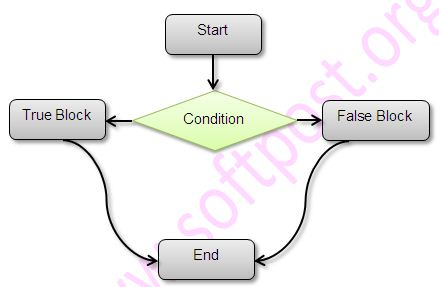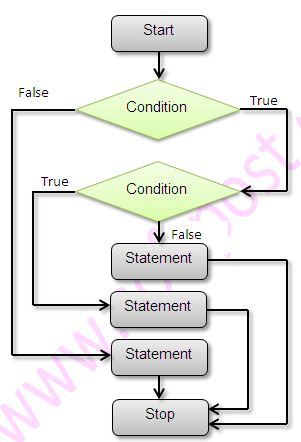There are 2 types of conditional statements in Java.
• if…..else
• Conditional operator ?:
• switch
Below Java example illustrates how we can use conditional constructs in Java.
``````
package conditional_statements;

/**
* Created by Sagar on 16-04-2016.
*/
public class Conditionals {

public static void main(String [] args){
int a=13,b=11,c=2;

if (a > b){
System.out.println("a is bigger than b");
}else {
System.out.println("a is less than b");
}

int choice=2;

switch(choice){
case 1:
System.out.println("Choice is " + choice);break;
case 2:
System.out.println("Choice is " + choice);
System.out.println("This block of code will be executed");
break;
case 3:
System.out.println("Choice is " + choice);break;
default:
System.out.println("Choice is default");
}

}
}
``````
Here is the output of above code.
``````
a is bigger than b
Choice is 2
This block of code will be executed
``````### Conditional Operator ?:

Below example illustrates use of conditional operator.
``````
package conditional_statements;

/**
* Created by Sagar on 19-04-2016.
*/
public class Condition {
public static void main(String args []){

int a = 10;
//normal if condition
boolean result ;
if (a%2==0){
result = true;
}else{
result = false;
}

System.out.println("Result is -> " + result);

//based on outcome of condition, assignment is done
result = (a%2==0) ? true :false;

System.out.println("Result is -> " + result);

}
}
``````
Here is the output of above code.
``````
Result is -> true
Result is -> true
``````Web development and Automation testing

solutions delivered!!